﻿ 电力设备铭牌特征提取方法的应用研究

# 电力设备铭牌特征提取方法的应用研究Application Research of Feature Extraction Method of Power Equipment Nameplate

Abstract: In the feature extraction problem of power equipment nameplate image, because of its similar underlying features, traditional feature extraction methods often fail to obtain better feature representation. On the other hand, with the development of invariant feature extraction methods in recent years, the feature algorithm based on multi-scale space represented by SIFT algorithm has a good performance in feature representation. In this paper, the SIFT method and its improved SURF and ORB algorithms are applied to the extraction of power equipment nameplate features. The ex-perimental results show that SURF is the most suitable feature extraction algorithm for power equipment nameplate images.

1. 引言

2. 尺度不变特征变换算法

David G. Lowe教授经过多年的研究，在2004年提出基于尺度不变特征变换(Scale Invariant Feature Transform, SIFT)算法，该算法将提取特征的过程分为四个步骤：在尺度空间上检测极值点、精确定位关键点位置、计算关键点主方向、计算特征点描述子。SIFT算法在实现原理上，最关键的部分就是在图像中构建多尺度空间，利用高斯金字塔寻找对尺度、光照、旋转等变化不敏感的特征点，使得提取的特征点具有较强的鲁棒性 。

2.1. 尺度空间极值点检测

$G\left(x,y,\sigma \right)=\frac{1}{2\pi {\sigma }^{2}}\mathrm{exp}\left(-\frac{{\left(x-{x}_{i}\right)}^{2}+{\left(y-{y}_{i}\right)}^{2}}{2{\sigma }^{2}}\right)$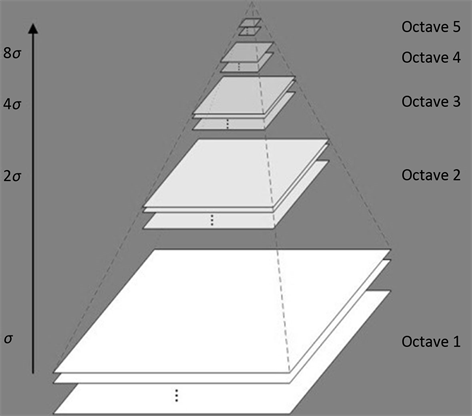Figure 1. Gaussian scale-space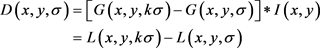2.2. 精确定位特征点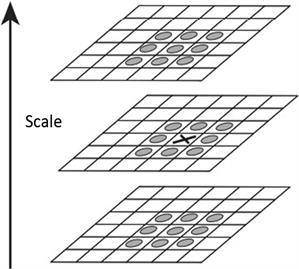Figure 2. Extreme point detection in Gaussian differential scale-space

$H=\left[\begin{array}{cc}{D}_{XX}& {D}_{XY}\\ {D}_{XY}& {D}_{YY}\end{array}\right]$

$\alpha =\gamma \beta$

$Tr\left(H\right)={D}_{XX}+{D}_{YY}=\alpha +\beta$

$Det\left(H\right)={D}_{XX}\cdot {D}_{YY}-{\left({D}_{YY}\right)}^{2}=\alpha ×\beta$

$\frac{Tr\left(H\right)}{Det\left(H\right)}=\frac{\alpha +\beta }{\alpha ×\beta }=\frac{{\left(\gamma +1\right)}^{2}}{\gamma }$

$\alpha$$\beta$ 的比值越大时， $Tr\left(H\right)$$Det\left(H\right)$ 的比值最大，此时说明图像在某个方向上梯度值与另一梯度值的差值越大，这种情况正好符合图像边缘的特征特点，为了去除特征点的边缘响应，需要将 $\gamma$ 设置一个阈值范围，并对上式做进一步判断 ：

$\frac{Tr\left(H\right)}{Det\left(H\right)}<\frac{{\left(\gamma +1\right)}^{2}}{\gamma }$

Lowe在论文中认为 $\gamma$ 取值为10能得到最好的效果。

2.3. 计算特征主方向

SIFT在计算特征主方向上，首先选特征点所在的尺度大小为 $3\sigma$ 的邻域范围内像素点集，计算邻域像素点的梯度幅度和梯度方向，具体计算公式如下所示，其中 $L\left(x,y\right)$ 表示图像中特征点所在尺度的空间值。

$gradI\left(x,y\right)=\left(\frac{\partial I}{\partial x},\frac{\partial I}{\partial y}\right)$

$m\left(x,y\right)=\sqrt{{\left[L\left(x+1,y\right)-L\left(x-1,y\right)\right]}^{2}+{\left[L\left(x,y+1\right)-L\left(x,y-1\right)\right]}^{2}}$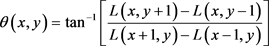Figure 3. Sketch map of selecting direction of feature points

2.4. 计算特征描述子

SIFT特征描述子是在DoG尺度空间中统计特征点的周围像素梯度得到，具体过程是：首先划分特征点邻近的像素为若干个子区域，并计算和统计每块子区域的梯度直方图，最终将每个直方图的结果组成一个特征表达向量。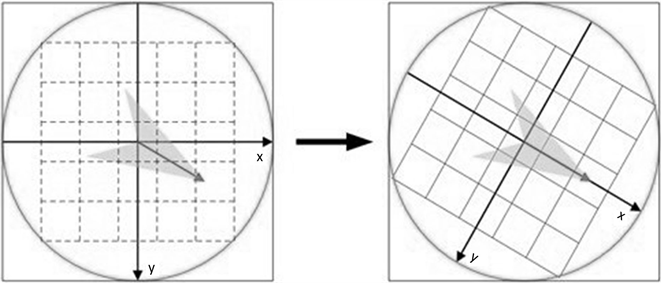Figure 4. Rotation of coordinate system to main direction of feature point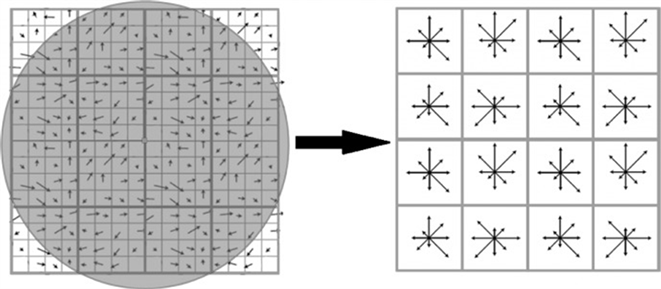Figure 5. Expression process of SIFT descriptor

3. 改进的SIFT算法

SIFT虽然能够在一定程度上保证了图像特征的不变性，但是仍然存在一定的不足，比如计算过程复杂、特征描述子的向量过大等问题。为了解决SIFT算法的不足，后来许多研究学者提出了改进的方案，其中SURF和ORB算法是其中较为成熟的改进方法。

3.1. SURF算法

Herbert Bay教授在2006年提出加速鲁棒特征(Speeded-Up Robust Features, SURF)算法，该算法是基于SIFT算法的改进。SURF算法在实现原理上与SIFT大致相同，在保持了SIFT算法中对尺度、光照、旋转、仿射等变化鲁棒性强的特点的同时，针对SIFT算法在设计中存在耗时长、特征描述子维度过大的问题进行了优化。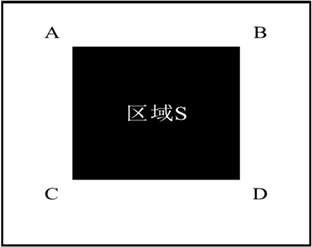Figure 6. Computation of S area in the image

3.1.1. 积分图像

SURF算法利用过了积分图像能够快速得到图像中任意区域的像素和。以图6为例，利用积分图像的公式可以快速得到区域S的像素值之和，其计算过程如下式所示。

${I}_{\text{Σ}}\text{S}=A+D-B-C$

3.1.2. 方格滤波器

SURF算法在构建尺度空间过程中，采用了方格滤波器来替代Gaussian滤波器，图7图8对比了在二维平面上，两种滤波器设计的不同。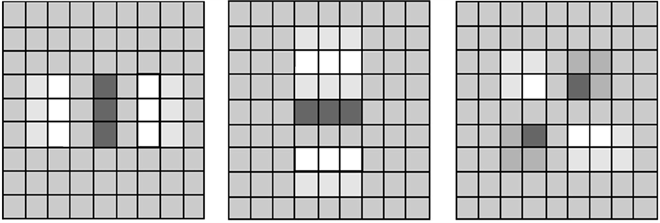(a) X方向 (b) Y方向 (c) XY方向

Figure 7. Second order Gaussian filter $L\left(X,\sigma \right)$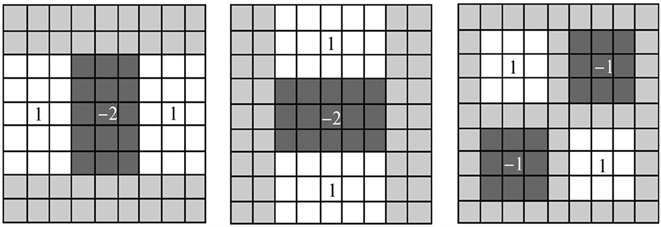(a) X方向 (b) Y方向 (c) XY方向

Figure 8. Second order grid filter

3.1.3. Haar小波

Haar小波是一种结构简单，且能够检测水平和垂直方向的梯度变化的滤波器。SURF利用图9(a)的Haar滤波器计算图像在水平方向的梯度，(b)计算垂直方向的梯度。

SURF算法利用Haar小波计算特征点邻域的Haar响应结果作为其特征方向，另外在计算特征描述子过程中，SURF算法统计特征点周围的 $5\sigma ×5\sigma$ 区域的Haar小波系数，得到一个64维的特征向量。(a) 水平方向 (b) 垂直方向

Figure 9. Haar wavelet with different direction

3.2. ORB算法

ORB(Oriented FAST and Rotated BRIEF)算法是一种基于二进制描述子的特征检测算法，该算法结合了FAST算法和BRIEF算法两种算法并加以改进。

FAST的主要思想是：若某像素与其周围邻域内足够多的像素点相差较大，则该像素可能是角点。BRIEF是一种基于二进制编码的特征点描述方法，具有匹配迅速的特点。

ORB的改进思路为：在检测特征点过程中，ORB利用Harris角点来限定FAST角点；在计算特征描述子上利用强度质心(intensity centroids)方法，提高特征描述子在特征方向描述能力。因此ORB算法具备了出色的算法实时性，同时在图像特征表达上也具备一定的稳健性 。

3.2.1. 特征点检测

FAST角点检测是在检测点周围邻域上比较差值大小，如图10所示，检测点P比较其周围16个点的像素值差值，若有足够多的周围点与检测点的像素差值超过阈值，即上述16个点的差值的平均值，则认为该点为特征点。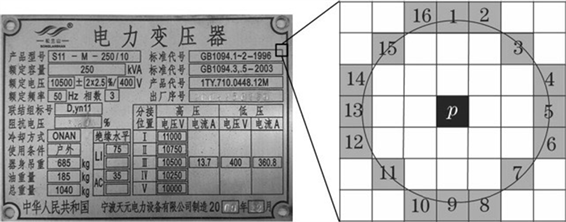Figure 10. Detecting feature points

ORB算法则增加了Harris检测对特征点进行排序，加入了响应函数，并在多尺度空间金字塔中计算FAST角点，增加了特征点的尺度不变性。

3.2.2. 计算特征主方向

ORB算法在计算特征主方向上，利用极值点与质心的灰度偏移量作为特征方向。假设特征点的邻域矩 ${M}_{p,q}$ 的计算公式为：

${M}_{p,q}={\sum }_{\left(x,y\right)\in \text{S}}{x}^{p}{y}^{q}I\left(x,y\right)$

$C=\left(\frac{{M}_{1,0}}{{M}_{0,0}},\frac{{M}_{0,1}}{{M}_{0,0}}\right)$

$\theta =\mathrm{arctan}\left({M}_{0,1},{M}_{1,0}\right)$

3.2.3. 计算特征描述子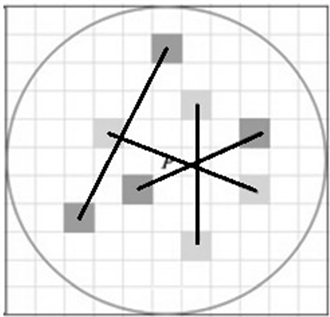Figure 11. Selecting point pairs based on BRIEF

${R}_{\theta }=\left(\begin{array}{cc}\mathrm{cos}\theta & \mathrm{sin}\theta \\ -\mathrm{sin}\theta & \mathrm{cos}\theta \end{array}\right)$

4. 电力设备铭牌特征提取实验

4.1. 实验数据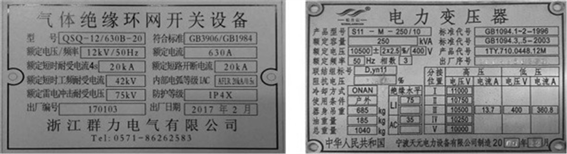Figure 12. Nameplate of electrical equipment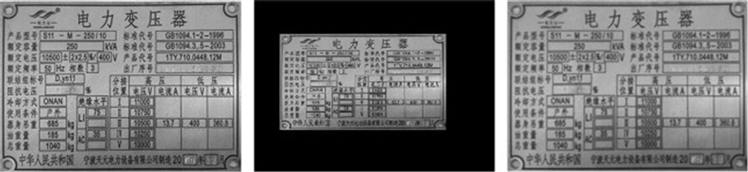(a) (b) (c)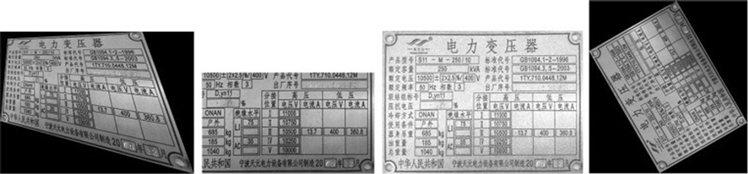(d) (e) (f) (g)

Figure 13. Change processing on nameplate of electrical equipment; (a) Original; (b) Zoom; (c) Slur; (d) Affine; (e) Cut; (f) Adding noise; (g) Rotate

4.2. 评价标准

4.2.1. 特征位置分散性及不变性

4.2.2. 特征提取效率

4.2.3. 正确率和召回率

4.3. 实验结果分析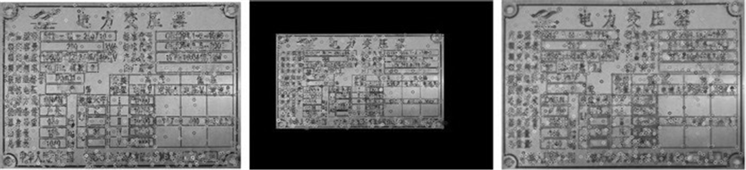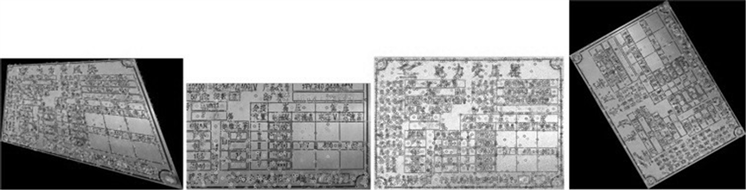Figure 14. Extracting feature points based on SIFT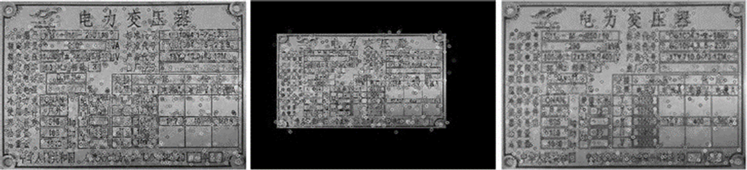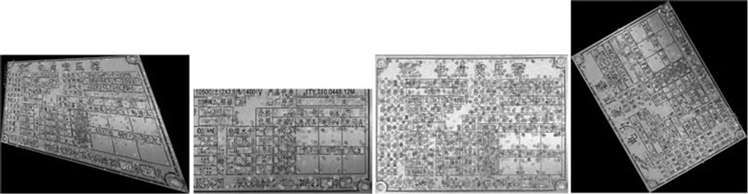Figure 15. Extracting feature points based on SURF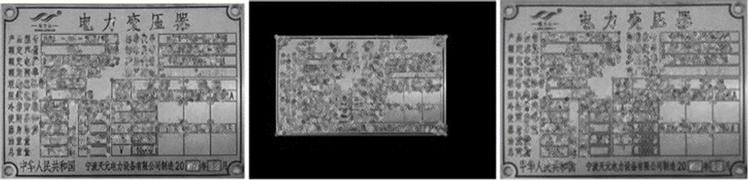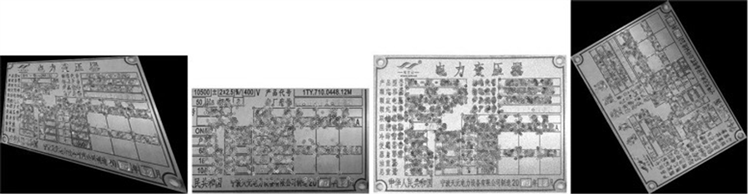Figure 16. Extracting feature points based on ORB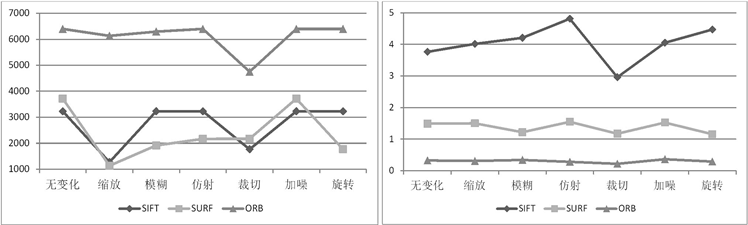(a) (b)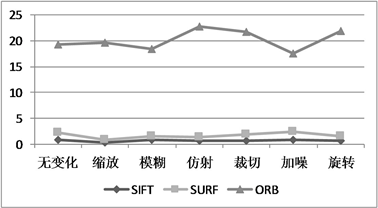(c)

Figure 17. Efficiency of extracting features with different algorithms; (a) Number of extracted features; (b) Time of extracting; (c) Number of extracted features in unit time(a)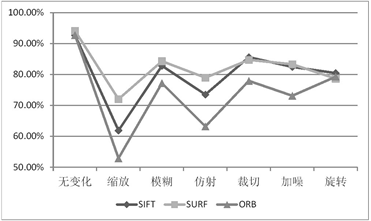(b)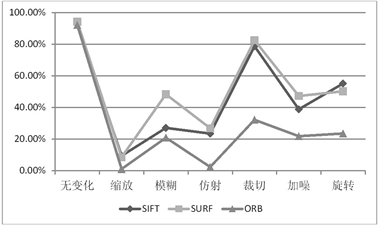(c)

Figure 18. Features matching experiments based on SIFT, SURF, and ORB; (a) Number of matched pairs of points; (b) Accuracy; (c) Recall rate

5. 结语

SIFT算法由于其在检测图像特征上，具备较高的稳定性和鲁棒性，得到了广泛的应用。与此同时，许多研究学者在基于SIFT算法提出了改进的方法，以提高特征提取的效率和性能。

 Lowe, D.G. (2004) Distinctive Image Features from Scale-Invariant Keypoints. International Journal of Computer Vi-sion, 60, 91-110.
https://doi.org/10.1023/B:VISI.0000029664.99615.94

 Yue, S.C., Wang, Q. and Zhao, R.C. (2009) Robust Wide Baseline Point Matching Based on Scale Invariant Feature Descriptor. Chinese Journal of Aero-nautics, 22, 70-74.
https://doi.org/10.1016/S1000-9361(08)60070-9

 Xu, Y.-P., Hu, K.-N., Tian, Y., et al. (2008) Classification of Hyper-Spectral Imagery Using SIFT for Spectral Matching. Proceedings of the 1st International Conference on Image and Signal Processing, Sanya, 27-30 May 2008, 704-708.
https://doi.org/10.1109/CISP.2008.114

 林传力, 赵宇明. 基于Sift特征的商标检索算法[J]. 计算机工程, 2008, 34(23): 275-277.

 洪志令. 基于形状匹配的商标图像检索技术研究[D]: [博士学位论文]. 厦门: 厦门大学, 2008.

 周明全, 耿国华, 韦娜. 基于内容图像检索技术[M]. 北京: 清华出版社, 2007.

 孙剑, 徐宗本. 计算机视觉中的尺度空间方法[J]. 工程数学学报, 2005(6): 5-16.

 张俭嘉. 视觉分类及其在场景分析中的应用[D]: [硕士学位论文]. 南京: 东南大学, 2012.

 张华贵. 基于局部关键点特征的视频近重复检测算法研究[D]: [硕士学位论文]. 上海: 复旦大学, 2012.

 Dai, X.B., Zhang, H., Shu, H.Z. and Luo, L.M. (2010) Image Recognition by Combined Invariants of Legendre Moment. IEEE International Conference on Information and Automa-tion, Harbin, 20-23 June 2010, 1793-1798.

 Bin, Y. and Xiong, P.J. (2002) Improvement and Invariance Analysis of Zernike Moments. International Conference on Communications, Circuits and Systems and West Sino Expositions, Chengdu, 29 June-1 July 2002, 963-967.

 Bay, H., Ess, A., Tuytelaars, T. and Van Gool, L. (2007) Speeded-Up Robust Features (SURF). Computer Vision and Image Understanding, 110, 346-359.
https://doi.org/10.1016/j.cviu.2007.09.014

 Rublee, E., Rabaud, V., Konolige, K. and Bradski, G. (2011) ORB: An Efficient Alternative to SIFT or SURF. IEEE International Conference on Computer Vision, Barcelona, 6-13 No-vember 2011, 2.
https://doi.org/10.1109/ICCV.2011.6126544

 Rosin, P.L. (1999) Measuring Corner Properties. Computer Vi-sion and Image Understanding, 73, 291-307.
https://doi.org/10.1006/cviu.1998.0719

Top